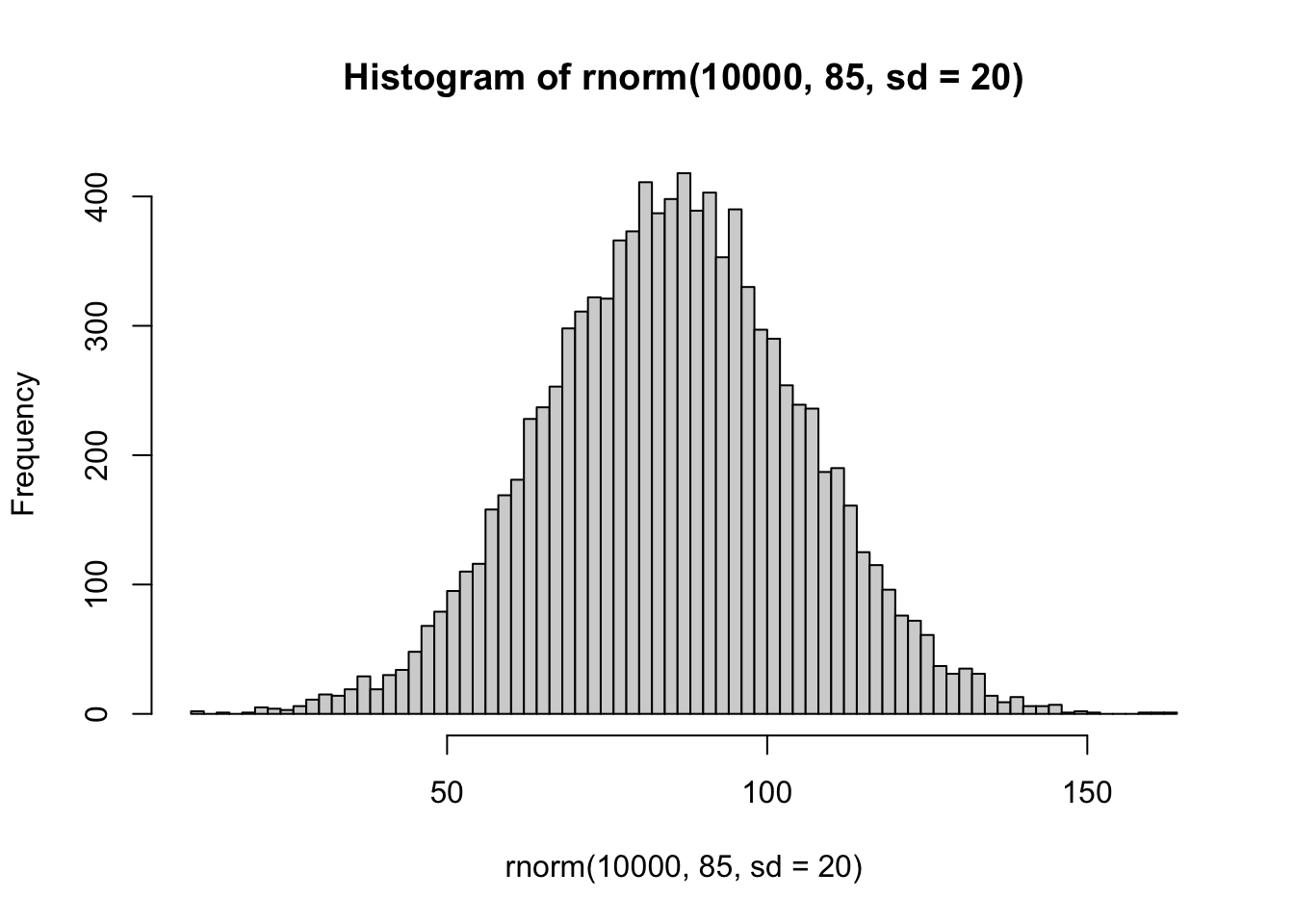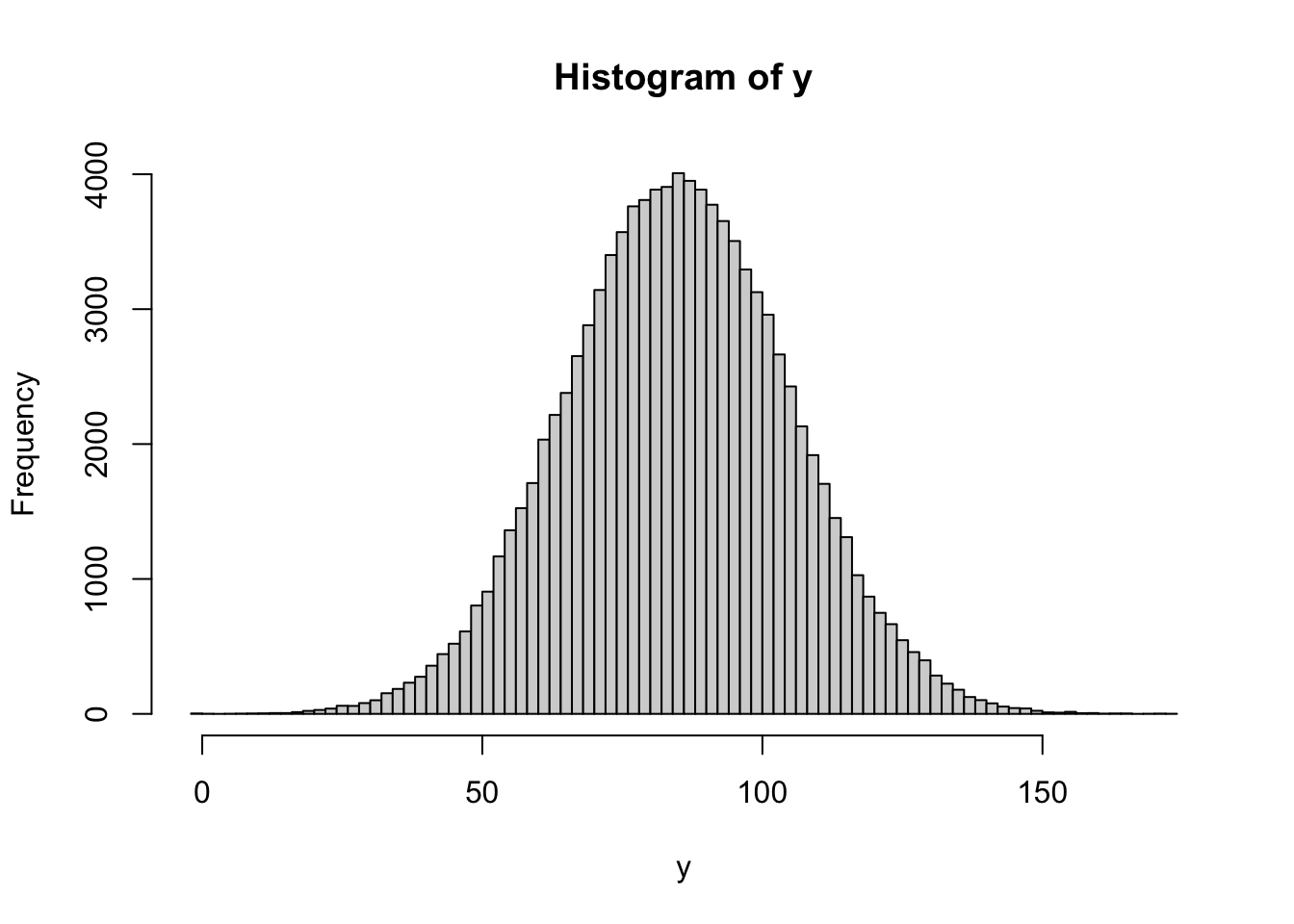Author

Gibran Hemani

Published

March 24, 2023

## Background

• People with high LDL cholesterol tend to take medication that lowers their observed LDL
• How does this affect genetic associations?

Get a rough distribution of LDL cholesterol (e.g. like this)``library(dplyr)``
``````
Attaching package: 'dplyr'``````
``````The following objects are masked from 'package:stats':

filter, lag``````
``````The following objects are masked from 'package:base':

intersect, setdiff, setequal, union``````
``hist(rnorm(10000, 85, sd=20), breaks=100)``Generate genetic effect for LDL cholesterol with effect `b_gy`

``````n <- 100000
g <- rnorm(n)
mean_ldl <- 85
sd_ldl <- 20
b_gy <- 10
y <- mean_ldl + g * b_gy + rnorm(n, sd=sqrt(sd_ldl^2 - b_gy^2))
hist(y, breaks=100)``````People with high LDL cholesterol more likely to take medication - make an adjusted LDL cholesterol measure which has a reduced value amongst people taking medications

``````n <- 100000
g <- rnorm(n)
mean_ldl <- 130
sd_ldl <- 20
b_gy <- 1
med_effect <- 0.8

y <- mean_ldl + g * b_gy + rnorm(n, sd=sqrt(sd_ldl^2-b_gy^2))
med <- rbinom(n, 1, plogis(scale(y)))
y_obs <- y
y_obs[as.logical(med)] <- y_obs[as.logical(med)] * med_effect

rbind(
summary(lm(y ~ g))\$coef[2,],
summary(lm(y_obs ~ g))\$coef[2,]
) %>% as_tibble() %>% mutate(measure=c("y", "y_obs"))``````
``````# A tibble: 2 × 5
Estimate `Std. Error` `t value` `Pr(>|t|)` measure
<dbl>        <dbl>     <dbl>      <dbl> <chr>
1    0.909       0.0632      14.4   7.62e-47 y
2    0.569       0.0552      10.3   6.46e-25 y_obs  ``````

What happens if you put in an approximate adjustment of y_obs. e.g. the effect of the drug is relative (20% reduction), we could erroneously add an absolute value onto med users to try to adjust

``````n <- 100000
g <- rnorm(n)
mean_ldl <- 130
sd_ldl <- 20
b_gy <- 1
med_effect <- 0.8

y <- mean_ldl + g * b_gy + rnorm(n, sd=sqrt(sd_ldl^2-b_gy^2))
med <- rbinom(n, 1, plogis(scale(y)))
y_obs <- y
y_obs[as.logical(med)] <- y_obs[as.logical(med)] * med_effect

rbind(
summary(lm(y ~ g))\$coef[2,],
summary(lm(y_obs ~ g))\$coef[2,],
``````# A tibble: 4 × 5
Estimate `Std. Error` `t value` `Pr(>|t|)` measure
<dbl>        <dbl>     <dbl>      <dbl> <chr>
1    0.936       0.0631      14.8   1.07e-49 y
2    0.602       0.0547      11.0   3.95e-28 y_obs
3    0.936       0.0631      14.8   1.07e-49 y_adj_true
4    0.789       0.0538      14.7   1.41e-48 y_adj_approx``````

Does collider bias have an impact? Statins are administered due to having a cardio event or being high risk e.g. due to family history. So there could be other non-LDL genetic factors that influence medication usage, and selecting or adjusting for medication usage could induce a collider that associates non-LDL genotypes with the adjusted LDL phenotype. e.g. simulate a large non-LDL factor that influences medication for illustration

``````n <- 1000000
g <- rnorm(n)
g_other <- rnorm(n)
mean_ldl <- 130
sd_ldl <- 20
b_gy <- 1
b_omed <- 1
med_effect <- 0.8

y <- mean_ldl + g * b_gy + rnorm(n, sd=sqrt(sd_ldl^2-b_gy^2))
med <- rbinom(n, 1, plogis(scale(y) + g_other * b_omed))
y_obs <- y
y_obs[as.logical(med)] <- y_obs[as.logical(med)] * med_effect

Result for LDL genotype

``````rbind(
summary(lm(y ~ g))\$coef[2,],
summary(lm(y_obs ~ g))\$coef[2,],
``````# A tibble: 4 × 5
Estimate `Std. Error` `t value` `Pr(>|t|)` measure
<dbl>        <dbl>     <dbl>      <dbl> <chr>
1    1.03        0.0200      51.5  0         y
2    0.680       0.0181      37.6  5.73e-309 y_obs
3    1.03        0.0200      51.5  0         y_adj_true
4    0.871       0.0172      50.5  0         y_adj_approx``````

Result for non-LDL genotype

``````rbind(
summary(lm(y ~ g_other))\$coef[2,],
summary(lm(y_obs ~ g_other))\$coef[2,],
``````# A tibble: 4 × 5
Estimate `Std. Error` `t value` `Pr(>|t|)` measure
<dbl>        <dbl>     <dbl>      <dbl> <chr>
1  -0.0168       0.0200    -0.841      0.401 y
2  -4.72         0.0175  -270.         0     y_obs
3  -0.0168       0.0200    -0.841      0.401 y_adj_true
4  -1.10         0.0172   -63.8        0     y_adj_approx``````

### Summary

• A relative reduction in observed LDL measures due to medication will lead to attenuated genetic effect estimates
• Adjustment to correct LDL values amongst medication users potentially resolves the issue
• Even an inaccurate adjustment can improve the estimate
• Collider bias induces a negative association between unadjusted LDL and non-LDL genetic factors, but not a great deal of issue at the LDL locus
• Perfect adjustment of medication would resolve this problem
• Imperfect adjustment partially avoids the issue but some bias remains at the non-LDL genetic factor

``sessionInfo()``
``````R version 4.2.3 Patched (2023-03-15 r84020)
Platform: aarch64-apple-darwin20 (64-bit)
Running under: macOS Monterey 12.6.2

Matrix products: default
BLAS:   /Library/Frameworks/R.framework/Versions/4.2-arm64/Resources/lib/libRblas.0.dylib
LAPACK: /Library/Frameworks/R.framework/Versions/4.2-arm64/Resources/lib/libRlapack.dylib

locale:
 en_GB.UTF-8/en_GB.UTF-8/en_GB.UTF-8/C/en_GB.UTF-8/en_GB.UTF-8

attached base packages:
 stats     graphics  grDevices utils     datasets  methods   base

other attached packages:
 dplyr_1.0.10

loaded via a namespace (and not attached):
 knitr_1.41        magrittr_2.0.3    tidyselect_1.2.0  R6_2.5.1
 rlang_1.0.6       fastmap_1.1.0     fansi_1.0.3       stringr_1.5.0
 tools_4.2.3       xfun_0.36         utf8_1.2.2        cli_3.5.0
 DBI_1.1.3         htmltools_0.5.4   assertthat_0.2.1  yaml_2.3.6
 digest_0.6.31     tibble_3.1.8      lifecycle_1.0.3   htmlwidgets_1.5.4
 vctrs_0.5.1       glue_1.6.2        evaluate_0.19     rmarkdown_2.16
 stringi_1.7.8     compiler_4.2.3    pillar_1.8.1      generics_0.1.3
 jsonlite_1.8.4    pkgconfig_2.0.3  ``````